# Data Visualisation in Graphics Using Python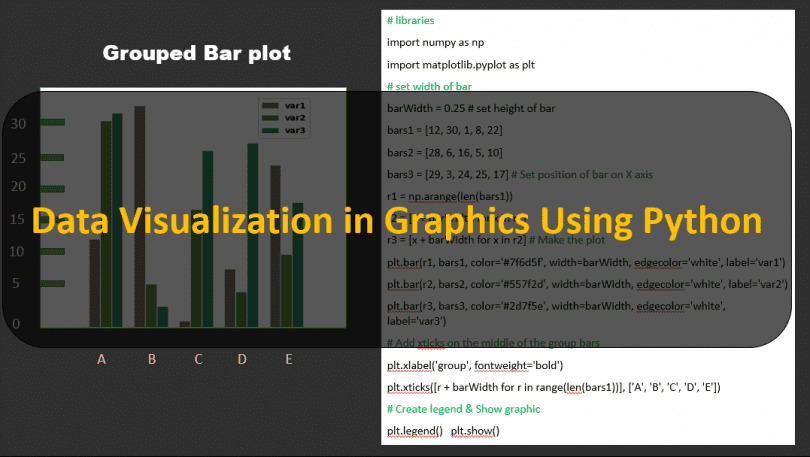In This tutorial You will learn about Graphs , How to plot a Graphs , Bar-chart, Box Plot , Venn Diagram , Area Chart , world cloud , Histogram Scatter plot  in Python we have Develop lots of Graphs with the help of Python for you and sharing Source code with you Guys.

Basic Bar Plot

Bar Plot is very common type of Graphs it use to Show Data with relationship like if we want to show Height and weight.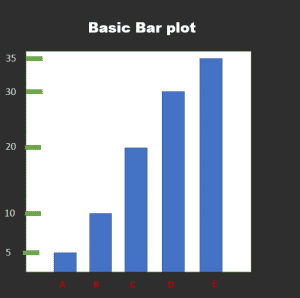Code

 ```import numpy as np import matplotlib.pyplot as plt >> Make a Your own Required dataset: height = [5, 10, 20, 30, 35] bars = ('A', 'B', 'C', 'D', 'E') y_pos = np.arange(len(bars)) >> Create bars plt.bar(y_pos, height) >> Create names on the x-axis plt.xticks(y_pos, bars) >> Show graphic plt.show()```

Area Plot Chart In Python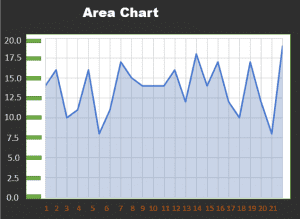Code of Area chart in python

 ```# libraries import numpy as np import seaborn as sns import matplotlib.pyplot as plt sns.set_style("whitegrid") # Color palette blue, = sns.color_palette("muted", 1) # Create data x = np.arange(23) y = np.random.randint(8, 20, 23) # Make the plot fig, ax = plt.subplots() ax.plot(x, y, color=blue, lw=3) ax.fill_between(x, 0, y, alpha=.3) ax.set(xlim=(0, len(x) - 1), ylim=(0, None), xticks=x) ```

Grouped Bar-plots in Python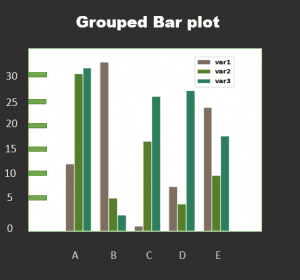Code of Grouped bar Plot in python

 ```# libraries import numpy as np import matplotlib.pyplot as plt # set width of bar barWidth = 0.25 # set height of bar bars1 = [12, 30, 1, 8, 22] bars2 = [28, 6, 16, 5, 10] bars3 = [29, 3, 24, 25, 17] # Set position of bar on X axis r1 = np.arange(len(bars1)) r2 = [x + barWidth for x in r1] r3 = [x + barWidth for x in r2] # Make the plot plt.bar(r1, bars1, color='#7f6d5f', width=barWidth, edgecolor='white', label='var1') plt.bar(r2, bars2, color='#557f2d',width=barWidth, edgecolor='white', label='var2') plt.bar(r3, bars3, color='#2d7f5e', width=barWidth, edgecolor='white', label='var3') # Add xticks on the middle of the group bars plt.xlabel('group', fontweight='bold') plt.xticks([r + barWidth for r in range(len(bars1))], ['A', 'B', 'C', 'D', 'E']) # Create legend & Show graphic plt.legend() plt.show() ```

Basic Venn Diagram with two groups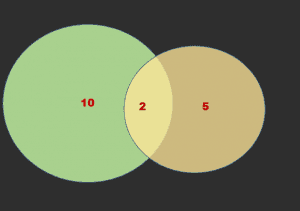Code of Basic Venn Diagram with 2 Groups  in python

 ` ` ```# library import matplotlib.pyplot as plt from matplotlib_venn import venn2 # First way to call the 2 group Venn diagram: venn2(subsets = (10, 5, 2), set_labels = ('Group A', 'Group B')) plt.show() # Second way venn2([set(['A', 'B', 'C', 'D']), set(['D', 'E', 'F'])]) plt.show()```